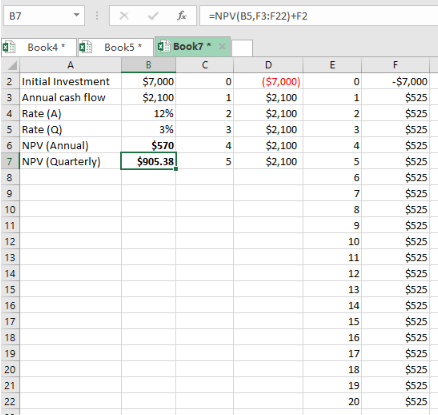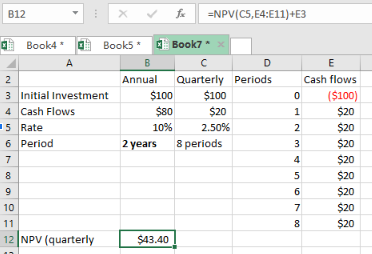Get instant live expert help with Excel or Google Sheets“My Excelchat expert helped me in less than 20 minutes, saving me what would have been 5 hours of work!”

#### Post your problem and you’ll get expert help in seconds.

Your message must be at least 40 characters
Our professional experts are available now. Your privacy is guaranteed.

# Learn How to Calculate NPV with Quarterly Cash Flows in Excel

By now it should be easy to calculate the NPV in excel when given annual cash flows. But what if we want to calculate the same but with quarterly cash flows? This post will provide a step-by-step guide on how to calculate NPV with quarterly cash flows in excel.Figure 1: How to find NPV when cash flows are quarterly

## General syntax of the formula

`=NPV (rate, cash flows) + Initial investment`

Where;

• Rate- will refer to the quarterly rate. We take annual rate multiply it by quarter
• Cash flows- refers to the number of quarters we have in the specified period. Take the periods multiply divide by quarter,

## Understanding the formula

The use of the NPV formula is fundamental when it comes to finding the NPV even when we have the cash flows as quarterly.

All we need to know is to understand how to make the rate and cash flow be quarterly, if they are given in annual terms.

## How the formula works

To calculate the net present value with a given initial investment and annual cash flows and annual rate, we need to first make the rate and time quarterly.

To do this, we multiply the rate by quarter and divide the time by quarter.

Ones we have these, we proceed to find the NPV the normal way using the formula;

`=NPV (rate, cash flows) + Initial investment`

## Example

Assuming that we have initial investment of \$100, and cash flows of \$80 for a period of 2 years, what will be the NVP given rate of interest as 10%?

We proceed as follows;

Step 1: Prepare and tabulate your Excel tableFigure 2: Example of how to find NPV with quarterly cash flows

Step 2: Prepare a column for the annual data and quarterly data as shown above.

Step 3: Enter the formula, with the quarterly data in the cell where you want to have the result for the NPV with quarterly cash flows.

Step 4: Press Enter to get the answer.

## Instant Connection to an Expert through our Excelchat Service

Most of the time, the problem you will need to solve will be more complex than a simple application of a formula or function. If you want to save hours of research and frustration, try our live Excelchat service! Our Excel Experts are available 24/7 to answer any Excel question you may have. We guarantee a connection within 30 seconds and a customized solution within 20 minutes.

Solution examplesI have a figure which is the sum of 9 months of payments. Each payment decreases by the same amount (X) so the first payment is Y, second payment is Y-X, third is Y-(Xx2), forth is Y-(Xx3) and so on. I am trying to calculate what each of the 9 payments should be.
Solved by A. W. in 60 mins